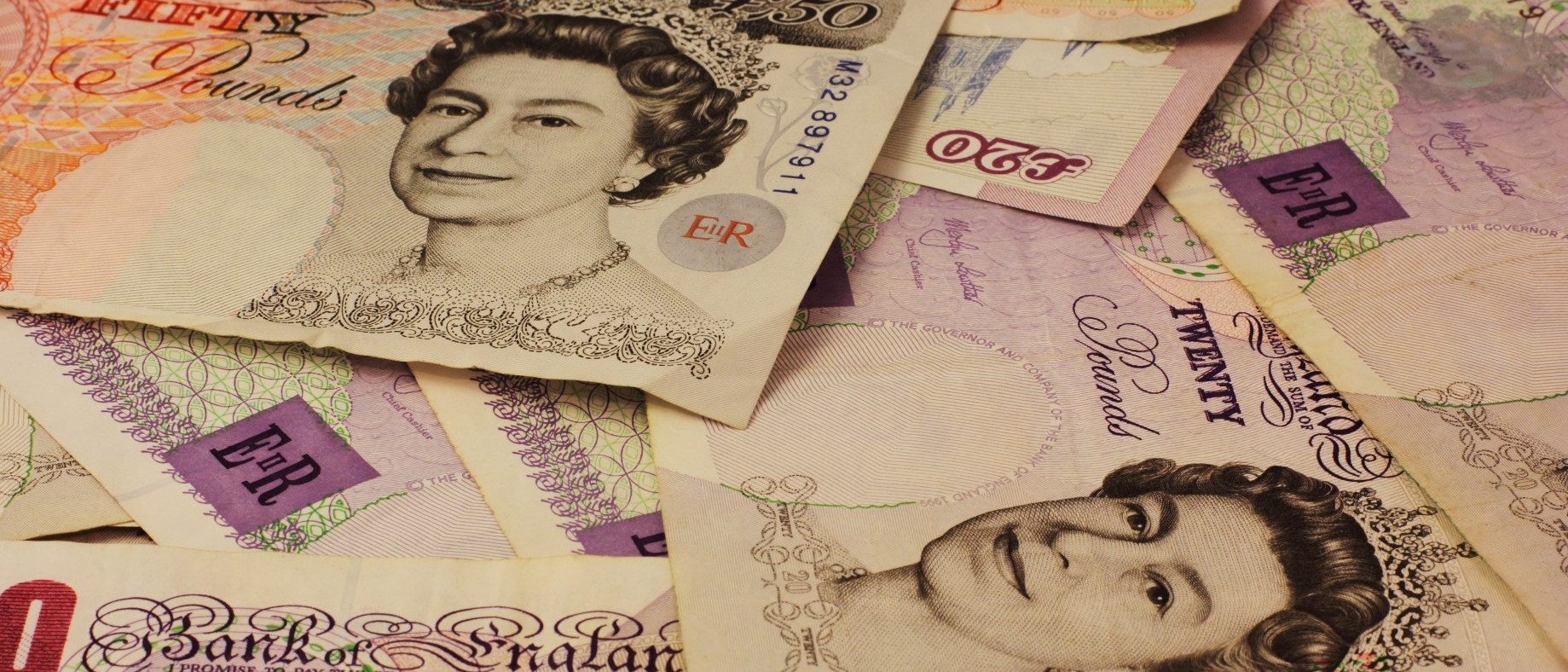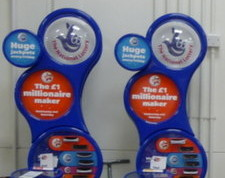# How much will I win on the new National Lottery?

What difference will an extra 10 balls make?From today, the National Lottery’s Lotto draw has 59 balls instead of 49. You may be thinking that this means there is now much less chance of winning. You would be right, except the prizes are also changing.

Camelot, who run the lottery, are saying that you are now “more likely to win a prize” and “more likely to become a millionaire”. But what do these changes actually mean?

### The Changes

Until yesterday, Lotto had 49 balls. From today, there are 59 balls. Each ticket still has six numbers on it and six numbers, plus a bonus ball, are still chosen by the lottery machine. The old prizes were as follows:

 Requirement Estimated Prize Match all 6 normal balls £2,000,000 Match 5 normal balls and the bonus ball £50,000 Match 5 normal balls £1,000 Match 4 normal balls £100 Match 3 normal balls £25 50 randomly picked tickets £20,000

The prizes have changed to:

 Requirement Estimated Prize Match all 6 normal balls £2,000,000 Match 5 normal balls and the bonus ball £50,000 Match 5 normal balls £1,000 Match 4 normal balls £100 Match 3 normal balls £25 Match 2 normal balls Free lucky dip entry in next Lotto draw One randomly picked ticket £1,000,000 20 other randomly picked tickets £20,000

### Probability of Winning a Prize

The probability of winning each of these prizes can be calculated. For example, the probability of matching all 6 balls in the new lotto is

$\mathbb{P}$(matching ball 1)$\times\mathbb{P}$(matching ball 2)$\times … \times\mathbb{P}$(matching ball 6)

$$=\frac{6}{59}\times\frac{5}{58}\times\frac{4}{57}\times\frac{3}{56}\times\frac{2}{55}\times\frac{1}{54}$$ $$=\frac{1}{45057474},$$
and the probability of matching 4 balls in the new lotto is
(number of different ways of picking four balls out of six)$\times\mathbb{P}$(matching ball 1)$\times … \times\mathbb{P}$(matching ball 4)$\times\mathbb{P}$(not matching ball 5)$\times\mathbb{P}$(not matching ball 6)

$$=15\times\frac{6}{59}\times\frac{5}{58}\times\frac{4}{57}\times\frac{3}{56}\times\frac{53}{55}\times\frac{52}{54}$$ $$=\frac{3445}{7509579}.$$ In the second calculation, it is important to include the probabilities of not matching the other balls to prevent double counting the cases when more than 4 balls are matched.Calculating a probability for every prize and then adding them up gives the probability of winning a prize. In the old draw, the probability of winning a prize was $0.0186$. In the new draw, it is $0.1083$. So Camelot are correct in claiming that you are now more likely to win a prize.

But not all prizes are equal: these probabilities do not take into account the values of the prizes. To analyse the actual winnings, we’re going to have to look at the expected amount of money you will win. But first, let’s look at Camelot’s other claim: that under the new rules you are more likely to become a millionaire.

### Probability of Winning £1,000,000In the old draw, the only way to win a million pounds was to match all six balls. The probability of this happening was $0.00000007151$ or $7.151\times 10^{-8}$.

In the new lottery, a million pounds can be won either by matching all six balls or by winning the millionaire raffle. This will lead to different probabilities of winning on Wednesdays and Saturdays due to different numbers of people buying tickets. Based on expected sales of 16.5 million tickets on Saturdays and 8.5 million tickets on Wednesdays, the chances of becoming a millionaire on a Wednesday or Saturday are $0.0000001398$ ($1.398\times 10^{-7}$) and $0.00000008280$ ($8.280\times 10^{-8}$) respectively.

These are both higher than the probability of winning a million in the old draw, so again Camelot are correct: you are now more likely to become a millionaire…

But the new chances of becoming a millionaire are actually even higher. The probabilities given above are the chances of winning a million in a given draw. But if two balls are matched, you win a lucky dip: you could win a million in the next draw without buying another ticket. We should include this in the probability calculated above, as you are still becoming a millionaire due to the original ticket you bought.

In order to count this, let $A_W$ and $A_S$ be the probabilities of winning a million in a given draw (as given above) on a Wednesday or a Saturday, let $B_W$ and $B_S$ be the probabilities of winning a million in this draw or due to future lucky dip tickets on a Wednesday or a Saturday (the values we want to find) and let $p$ be the probability of matching two balls. We can write $$B_W=A_W+pB_S$$ and $$B_S=A_S+pB_W$$ since the probability of winning a million is the probability of winning in this draw ($A$) plus the probability of winning a lucky dip ticket and winning in the next draw ($pB$). Substituting and rearranging, we get $$B_W=\frac{A_W+pA_S}{1-p^2}$$ and $$B_W=\frac{A_S+pA_W}{1-p^2}.$$

Using this (and the values of $A_S$ and $A_W$ calculated earlier) gives us probabilities of $0.0000001493$ ($1.493\times 10^{-7}$) and $0.00000009736$ ($9.736\times 10^{-8}$) of becoming a millionaire on a Wednesday and a Saturday respectively. These are both significantly higher than the probability of becoming a millionaire in the old draw ($7.151\times 10^{-8}$).

Camelot’s two claims—that you are more likely to win a prize and you are more likely to become a millionaire—are both correct. It sounds like the new lottery is a great deal, but so far we have not taken into account the size of the prizes you will win and have only shown that a very rare event will become slightly less rare. Probably the best way to measure how good a lottery is is by working out the amount of money you should expect to win, so let’s now look at that.

### Expected Prize Money

To find the expected prize money, we must multiply the value of each prize by the probability of winning that prize and then add them up, or, in other words, $$\sum_\mathrm{prizes}\mathrm{value\ of\ prize}\times\mathbb{P}(\mathrm{winning\ prize}).$$

Once this has been calculated, the chance of winning due to a free lucky dip entry must be taken into account as above.In the old draw, after buying a ticket for £2, you could expect to win 78p or 83p on a Wednesday or Saturday respectively. In the new draw, the expected winnings have changed to 58p and 50p (Wednesday and Saturday respectively). Expressed in this way, it can be seen that although the headline changes look good, the overall value for money of the lottery has significantly decreased.

Looking on the bright side, this does mean that the lottery will make even more money that it can put towards charitable causes: the lottery remains an excellent way to donate your money to worthy charities!Matthew is a postdoctoral researcher at the University of Cambridge. He hasn’t had time to play Klax since the noughties, but he’s pretty sure that Coke is it!
@mscroggs    mscroggs.co.uk    + More articles by Matthew